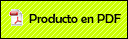# Foliation of the Phase Space for the Kepler Problem with Anisotropic Perturbations

###Criollo Pérez, Arturo2009

Arturo Criollo and Ernesto Pérez-Chavela. Foliation of the phase space for the Kepler Problem with anisotropic perturbations. Qualitative Theory of Dynamical Systems Vol. 7 No. 2 p. 435-449. 2009.

Abstract

We study a particular perturbation of the Kepler problem givenby the potential U(r, ?) = ?1/r ? b/r2(1 + cos2 ?), where b and are theperturbation parameters. This problem has two first integrals in involution:the first one is the well known Hamiltonian H = (p2r+p2?/r2)?1/r?b/r2(1+ cos2 ?); the second one is given by G = p2?/2 ? b/(1 + cos2 ?). The setsH?1(h), G?1(g) and H?1(h)G?1(g) are invariant under the flow of theHamiltonian system. From here we obtain a nice foliation of the phase space.In this paper we study the topology of the above foliation.Enseñanza de la Ley de Grashof con Cabri Geometry: Una Tarea de Aprendizaje

Mathematical thinking, conceptual frameworks: a review of structures for analyzing problema- solving...

International Journal of Pure and Applied Mathematics

FRACCIONES PARCIALES: ELEMENTOS PARA UNA DISCUSIÓN EN EL AULA